# How to subtract in Excel? Discover tricks and methods to do it easily

If you already know how to use the SUM function in Excel, by learning how to subtract in Excel you will be one step closer to becoming an expert and simplifying your tasks. Remember that this program is useful for countless activities.

According to the CERTUS Institute blog: “Excel allows you to create a schedule type scheme, manage budgets, track expenses and income, manage inventories, make charts, dashboards to represent data, create processes, etc.”.

However, before starting with the note, it is important to mention that the RESTA function in Excel does not exist. Therefore, you must consider other options to get to the result; but, don’t worry, it’s not a big deal!

Next, we will explain how to subtract in Excel, how to subtract several cells in Excel, how to do a subtraction in Excel from one sheet to another and other tricks to be able to do this simple mathematical operation in your spreadsheet.

## how to subtract in Excel?

You might be surprised to know that there are many ways to learn how to subtract in Excel. However,there is no one method that is better than another, in fact, the most suitable one for you depends exclusively on the tasks you have to perform.

In this section, you will learn 4 simple methods to learn how to do subtraction in Excel in various scenarios.

### 1. Writing numbers in a cell

This is the simplest way to subtract in Excel, although it will not help you in complex cases. Therefore, it is preferable that you use it to solve operations that are short and quick to type .

According to the official Microsoft Support page, to subtract cells in Excel you just have to place yourself in a cell and type the equal sign (=), followed by the values to be subtracted, which will be separated by the arithmetic operator, which is the minus sign (-).

You can try the following examples:

Click in a cell and type the formula: =10-5
Then press Enter and you will see the answer: 5

To do it with more numbers, just add them to the Excel subtraction formula:

Place this formula in a cell: =10-5-2
Press Enter and check that the answer is 3

As you can see, the first Excel subtraction method acts as a calculator in your spreadsheet. It will certainly help you perform quick operations to complement the analysis you are doing in your project.Image: Pexels

### 2. Using cell references

In the same way, you can use cell references to get to the result you want. In this case, the values to be subtracted in Excel have to be located in different cells and, in a separate cell, you will have to perform the operation.

The main benefit of subtracting in Excel with cell references in a formula is that you can change the values without having to modify the formula. In other words, it is ideal for building accounting tables or for preparing financial statements, since you can obtain automatic results just by entering different data.

In the following example, we are going to subtract in Excel the “Cost of production ” from the “Sales value of a product ” to calculate the “Profit ” or profit.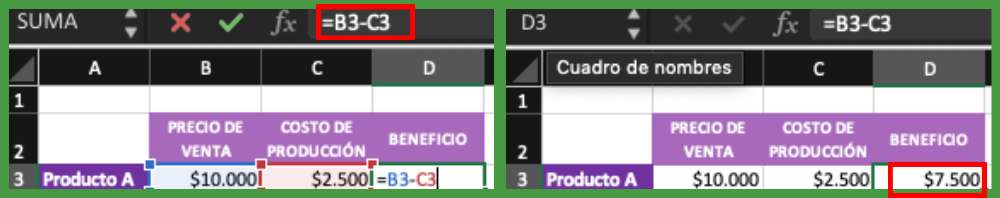Source: Excel

As you can see in the image, you only have to allocate a section of the document to place the totals -in this case it would be the “Profit” cell-, then, you have two options:

Write the arithmetic symbols and also the name of each cell, i.e.: =B3-C3
Type the arithmetic symbols and click on the cells that are part of the operation so that they are automatically included.

### 3. With the SUM function

“Excel functions are designed to save us time, because, through the predesigned formulas they handle, the program can operate automatically based only on some data”.

Therefore, using it to do a subtraction in Excel is really intuitive. The SUM function is a type of subtraction function in Excel -remember that the latter does not exist-, so you must accommodate it to get the result you expect.

To do this, you just need to add the negative sign next to the numbers you are going to include in the Excel subtraction formula, then click on an empty cell and assemble the SUM function with the values you want, follow this example:

=SUM(100,-32,15-6)

Then, press Enter and you will see the result: 77

### 4. By ranges

On the other hand, with the SUM function you can also do a subtraction in Excel by ranges; this will help you to subtract 3 or more columns in Excel.

Here is an example:

We have built a table in which we will have to subtract the “Selling price of Product A ” from the sum of the “Production cost ” with the value of the “Address” and the “Promotion discount” .

To practice how to do a subtraction in Excel by ranges, follow this path:

Open the formula with equal sign (=) and we quote the cell containing the value we are going to subtract from, which in this case would be B3.
Next, enter the arithmetic operator, that is, the minus sign (-).
Then, type the SUM function, inside which you must put the range containing the values to be subtracted: from cell C3 to E3.
The formula would look like this: =B3-SUM(C3:E3)
Press Enter and check that the result is \$6,000.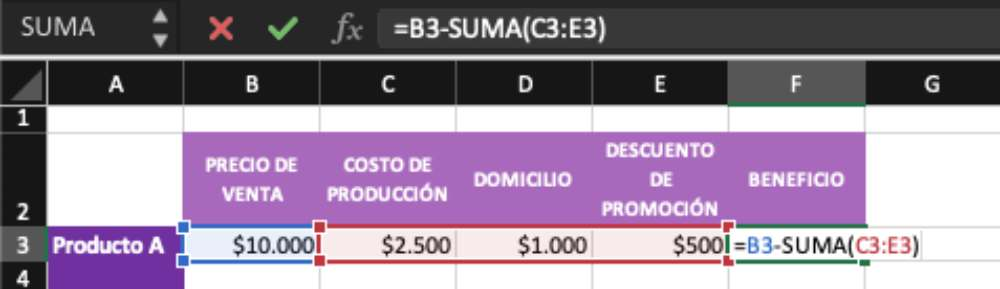Source: Excel

Another way that you may also find useful is the following: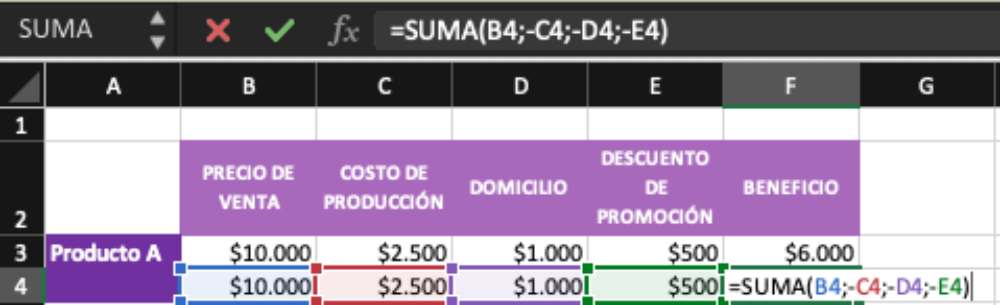Source: Excel

As you can see, you can enter each cell manually, separating them with the semicolon (;) and including the minus sign (-) before the value you are going to subtract in Excel.

Finally, you can also include the minus sign (-) in the numbers you want to subtract to save steps. Then, you must click on an empty cell and open the SUM function, select the range of cells you want to subtract in Excel and press Enter.

## how to do a subtraction in Excel from one sheet to another?

Now let’s learn how to subtract in Excel from one sheet to another. For this, it is necessary that the data is part of the same Excel file, so that you can tell the formula which spreadsheet each value belongs to.

To better explain this example, we will divide the data of the table we have been using in the previous exercises: in Sheet 1 will be the “Sales Price”, while, in Sheet 2, we will find the “Production Cost” and other values

In this opportunity, the objective is to calculate the “Profit ” of “Product A”, for which we will have to subtract in Excel the following operation: “Selling price” “Production cost”.

To do so, we consider the following steps:

Choose an empty cell and insert the function SUM(=SUM).
Then, select the cell we are going to subtract on Sheet 1: B2 or Sales Price .
Next, type the arithmetic operator, i.e. the minus sign (-).
Now, go to Sheet 2 and click on the “Cost of Production” value.
Close the function with parentheses and press Enter.

The formula for this example should look like this: =SUM(B2-Sheet2!B2)

that’s it! If you have followed our example, you should have \$7,500 as the result.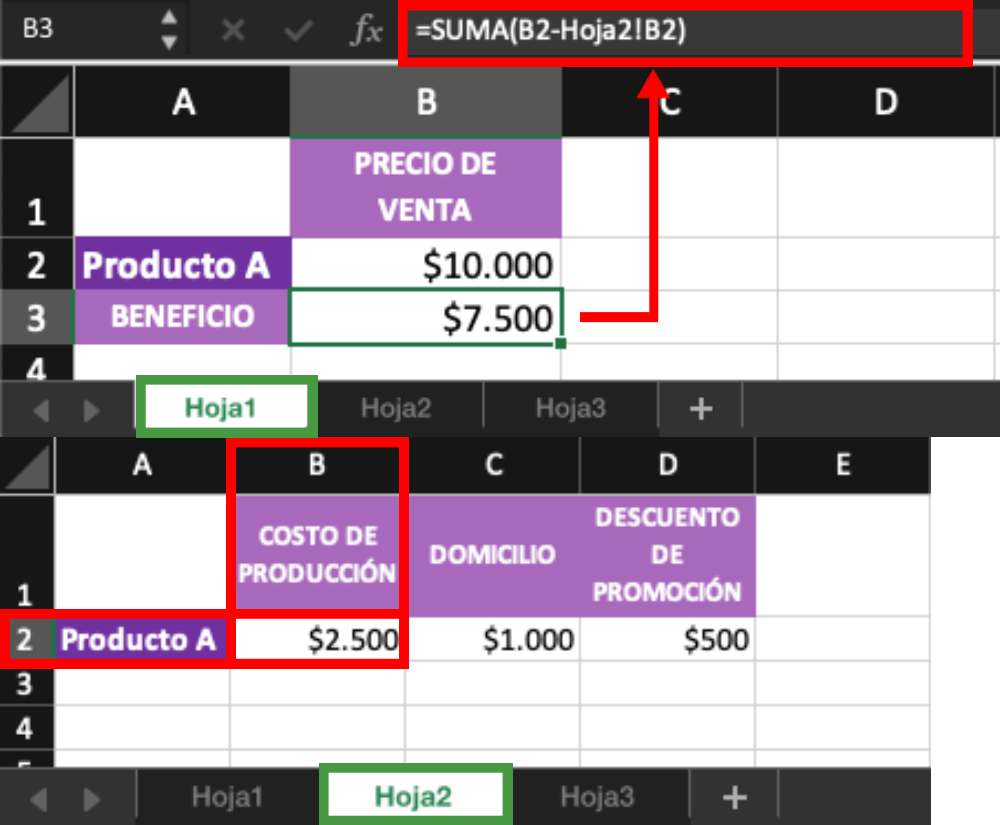Source: Excel

Now, if you want to subtract more data, just add, in the same way, the rest of the arguments with the minus sign (-), as you see in the following example, in which we also subtract the value of the “Address” and the “Promotion discount”:

The formula should look like this: =SUM(B2-Sheet2!B2-Sheet2!C2-Sheet2!D2).

And, as a result, \$6,000 is obtained.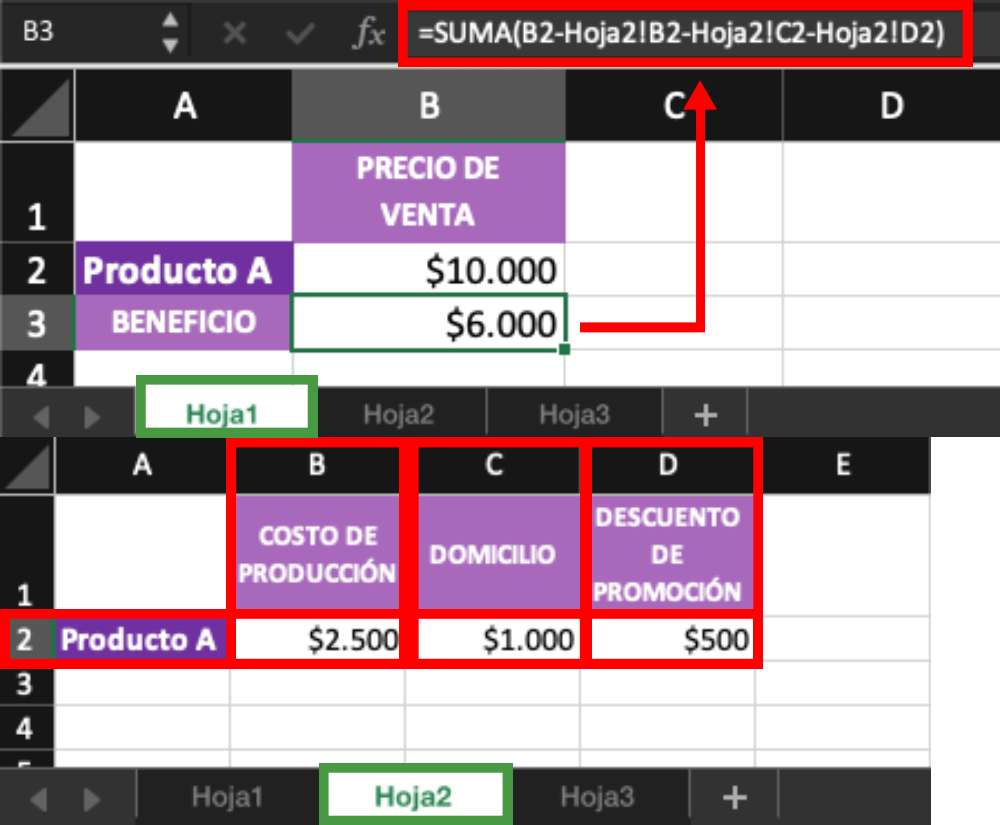Source: Excel

Remember that the possibilities in this program are endless.

good luck!